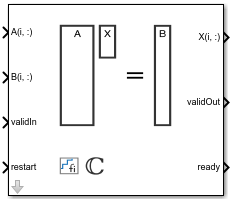# Complex Burst Matrix Solve Using QR Decomposition

Compute the value of x in the equation Ax = B for complex-valued matrices using QR decomposition

• Library:
• Fixed-Point Designer HDL Support / Matrices and Linear Algebra / Linear System Solvers

•## Description

The Complex Burst Matrix Solve Using QR Decomposition block solves the system of linear equations Ax = B using QR decomposition, where A and B are complex-valued matrices. To compute x = A-1, set B to be the identity matrix.

## Ports

### Input

expand all

Rows of matrix A, specified as a vector. A is an m-by-n matrix where m ≥ 2 and mn. If B is single or double, A must be the same data type as B. If A is a fixed point data type, A must be signed, use binary-point scaling, and have the same word length as B. Slope-bias representation is not supported for fixed-point data types.

Data Types: `single` | `double` | `fixed point`
Complex Number Support: Yes

Rows of matrix B, specified as a vector. B is an m-by-p matrix where m ≥ 2. If A is single or double, B must be the same data type as A. If B is a fixed-point data type, B must be signed, use binary-point scaling, and have the same word length as A. Slope-bias representation is not supported for fixed-point data types.

Data Types: `single` | `double` | `fixed point`
Complex Number Support: Yes

Whether inputs are valid, specified as a Boolean scalar. This control signal indicates when the data from the A(i,:) and B(i,:) input ports are valid. When this value is 1 (`true`) and the value at ready is 1 (`true`), the block captures the values at the A(i,:) and B(i,:) input ports. When this value is 0 (`false`), the block ignores the input samples.

After sending a `true` validIn signal, there may be some delay before ready is set to `false`. To ensure all data is processed, you must wait until ready is set to `false` before sending another `true` validIn signal.

Data Types: `Boolean`

Whether to clear internal states, specified as a Boolean scalar. When this value is 1 (`true`), the block stops the current calculation and clears all internal states. When this value is 0 (`false`) and the validIn value is 1 (`true`), the block begins a new subframe.

Data Types: `Boolean`

### Output

expand all

Rows of the matrix X, returned as a scalar or vector.

Data Types: `single` | `double` | `fixed point`

Whether the output data is valid, returned as a Boolean scalar. This control signal indicates when the data at the output port X(i,:) is valid. When this value is 1 (`true`), the block has successfully computed a row of matrix X. When this value is 0 (`false`), the output data is not valid.

Data Types: `Boolean`

Whether the block is ready, returned as a Boolean scalar. This control signal indicates when the block is ready for new input data. When this value is 1 (`true`) and validIn value is 1 (`true`), the block accepts input data in the next time step. When this value is 0 (`false`), the block ignores input data in the next time step.

After sending a `true` validIn signal, there may be some delay before ready is set to `false`. To ensure all data is processed, you must wait until ready is set to `false` before sending another `true` validIn signal.

Data Types: `Boolean`

## Parameters

expand all

Number of rows in input matrices A and B, specified as a positive integer-valued scalar.

#### Programmatic Use

 Block Parameter: `m` Type: character vector Values: positive integer-valued scalar Default: `4`

Number of columns in input matrix A, specified as a positive integer-valued scalar.

#### Programmatic Use

 Block Parameter: `n` Type: character vector Values: positive integer-valued scalar Default: `4`

Number of columns in input matrix B, specified as a positive integer-valued scalar.

#### Programmatic Use

 Block Parameter: `p` Type: character vector Values: positive integer-valued scalar Default: `1`

Data type of the output matrix X, specified as `fixdt(1,18,14)`, `double`, `single`, `fixdt(1,16,0)`, or as a user-specified data type expression. The type can be specified directly, or expressed as a data type object such as `Simulink.NumericType`.

#### Programmatic Use

 Block Parameter: `OutputType` Type: character vector Values: `'fixdt(1,18,14)'` | `'double'` | `'single'` | `'fixdt(1,16,0)'` | `''` Default: `'fixdt(1,18,14)'`

## Tips

Use `fixed.getMatrixSolveModel(A,B)` to generate a template model containing a Complex Burst Matrix Solve Using QR Decomposition block for complex-valued input matrices `A` and `B`.

## Extended Capabilities

Introduced in R2019b

## Support평가판 신청